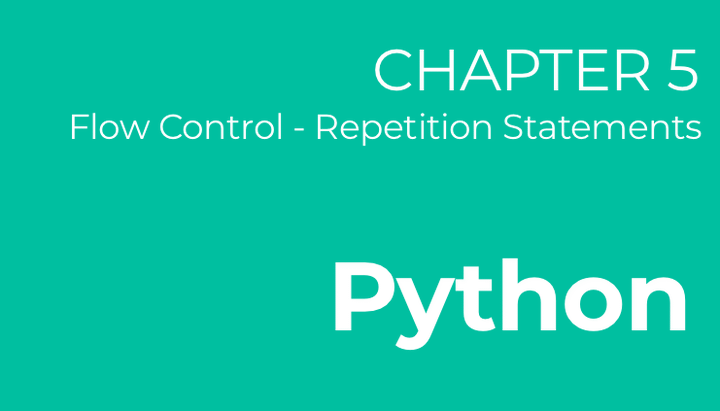# Python - Chapter 5

Flow Control (Repetition Statements)In chapter 4, we saw that we can control the flow of a program using selection statements - i.e. with the keywords `if`, `elif` and `else`. The next mode of flow control will be using repetition.

## The While Statement

The `while` statement allows us to repeatedly execute a block of code as long as a condition remains valid.

``````x = 3

while(x > 0):
print("Looping")
x -= 1	# equivalent to writing x = x - 1``````

The above code will print `Looping` for a total of 3 times before terminating. As you would have noticed, in each loop we decrement the value of `x` by one. The first time we execute the loop, `x` is 3. The second time it would be 2 and so on, until in the final loop when it becomes 0. Now, the next time we check if `x > 0`, the condition evaluates to be false and hence, the loop terminates.

If we try to represent the above code block in terms of a simple flowchart, it will look like this.

## The For Loop

We can also execute a code block repeatedly with the `for` loop.

``````# prints 0, 1, 2
for i in range(3):
print(i)

# prints 2, 3, 4, 5, 6
for i in range(2, 7):
print(i)

# prints 0, 2, 4, 6, 8
for i in range(0, 10, 2):
print(i)``````

The above three examples show how we can use `for` loops with the `range` operator.

In the first example, variable `i` gets assigned to 0 first. `range(3)` means that the loop is executed as long as `i < 3`. In every loop, `i` is incremented by 1.

Similarly, in the second example, `range(2, 7)`, `i` gets a starting value of 2 and the loop is executed as long as `i < 7`. In every loop, `i` is incremented by 1.

In the third example, `range(0 ,10, 2)`, i gets a starting value of 0 and the loop is executed as long as `i < 10`. While the first two example incremented `i` by 1 in each loop, here `i` is incremented by 2. This explains why the output is from 0 to 10 in multiples of 2.

## The Break Statement

Both `while` and `for` loops provide great utility in almost every program you write. Another useful statement is `break` which is used to exit out of a loop.

``````i = 0
while True:
print(i)
i += 1
if(i > 3):
break``````

In this example, we enter an infinite loop by saying `while True`. To break out of this infinite loop, we check for a specific condition if `i > 3` in every loop. If that condition evaluates to be true, we `break` out of the loop - i.e. loop is terminated.

## The Continue Statement

Sometimes we would also want to skip a specific iteration of the loop. We can do this using the `continue` statement. Previously, we saw that we can print all even numbers from 0 to 10 using `range(0, 10, 2)`. Lets try re-writing this, making use of the `continue` statement now.

``````for i in range(0, 10):
if(i % 2 != 0):
continue
print(i)``````

Here, in each iteration/loop, we check if `i` is odd by using the condition `i % 2 != 0`. This reads as "if the remainder when `i` is divided by 2 is not 0", we continue onto the next iteration without executing any of the following statements.

For example, when `i` is equivalent to 3, since (3 % 2 = 1), the condition `i % 2 != 0` evaluates to `false`. We continue onto the next iteration without executing line 4 - i.e. nothing is printed.I code.